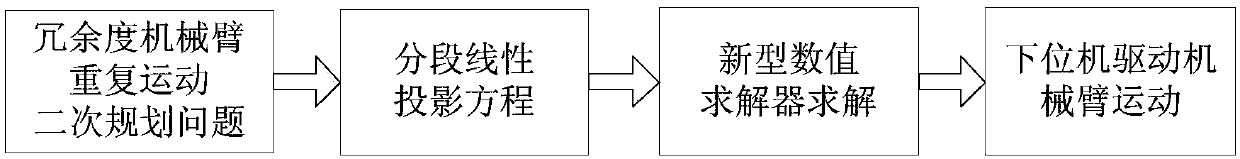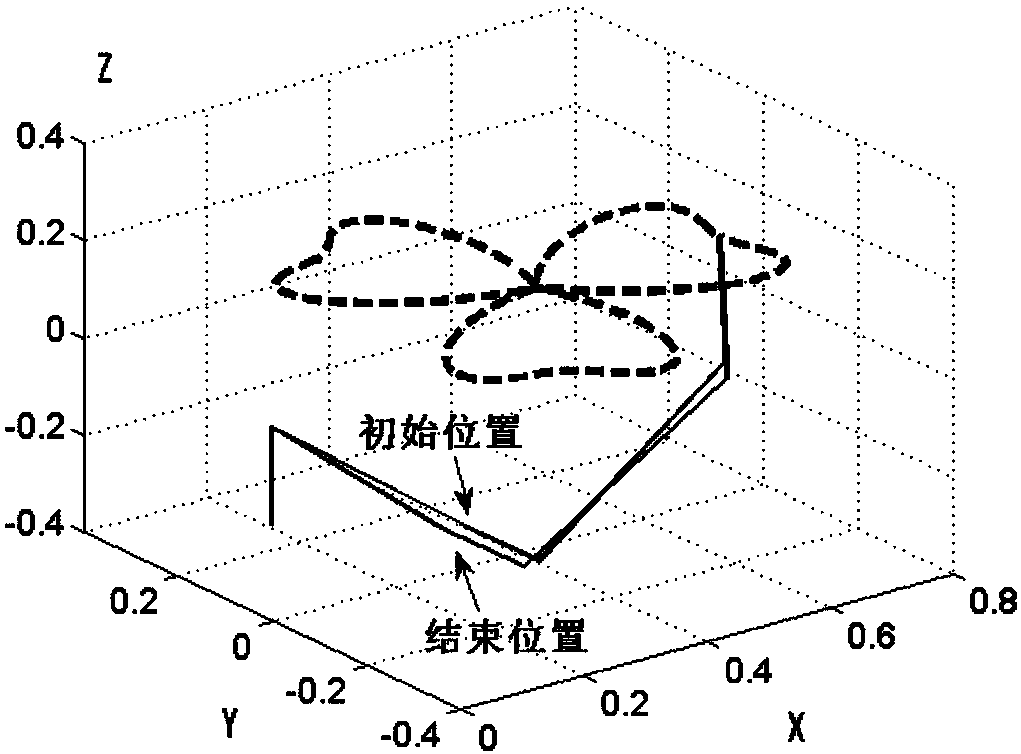# Method for planning repetitive motion of redundant manipulator based on new numerical solver

## A numerical solution and repetitive motion technology, applied in manipulators, program-controlled manipulators, instruments, etc., can solve problems such as difficult digital motor drive and control, and achieve the effect of reducing difficulty and simplifying the control process.

Inactive Publication Date: 2017-08-18
SOUTH CHINA UNIV OF TECH
2 Cites 14 Cited by

## AI-Extracted Technical Summary

### Problems solved by technology

However, most of the solution methods used in the existing redundant robotic arm motion planning methods are ti...
the structure of the environmentally friendly knitted fabric provided by the present invention; figure 2 Flow chart of the yarn wrapping machine for environmentally friendly knitted fabrics and storage devices; image 3 Is the parameter map of the yarn covering machine
View more

### Method used

Utilize numerical solver (12)-(15) to solve the piecewise linear projection equation (11) and need to give the initial position of mechanical arm in advance, set preset accuracy E and maximum number of iterations K, by constantly iterating operation to reduce errors. The operation ends when the second norm of the error ve...
the structure of the environmentally friendly knitted fabric provided by the present invention; figure 2 Flow chart of the yarn wrapping machine for environmentally friendly knitted fabrics and storage devices; image 3 Is the parameter map of the yarn covering machine
View more

## Abstract

The invention provides a method for planning repetitive motion of a redundant manipulator based on a new numerical solver. The method comprises the following steps of 1) taking a repetition performance index as a minimum performance index, and planning and designing repetitive motion of a redundant manipulator as a quadratic programming problem; 2) converting the quadratic planning problem in step 1) into a piecewise linear projection equation; 3) solving the piecewise linear projection equation in step 2) with a new numerical solver; and 4) transmitting the solution result in step 3) to a lower motor so as to drive a manipulator to move. According to the method for planning the repetitive motion of the redundant manipulator based on the new numerical solver, planning of the repetitive motion of the redundant manipulator is performed by adopting the new numerical solver based on the sectional piecewise linear projection equation so as to ensure that the manipulator can perform the repetitive motion without deviation; and a solving result is a discrete numerical, so that the difficulty for computer control and motor drive is reduced, and a control process is simplified.

Application Domain

Technology Topic

## Image

•••## Examples

• Experimental program(1)

### Example Embodiment

 The present invention will be further described below with reference to the accompanying drawings, but the implementation and protection of the present invention are not limited thereto.
 figure 1 The overall process shown is mainly composed of the quadratic programming problem of repetitive motion of the redundant manipulator, the piecewise linear projection equation, the solution of the new numerical solver, and the motion of the manipulator driven by the lower computer. Firstly, the repetitive motion planning of the redundant manipulator is designed as a quadratic programming problem on the velocity layer, and then the quadratic programming problem is transformed into a piecewise linear projection equation, and then a new numerical solver is used to solve the piecewise linear projection equation, and finally Transfer the solution results to the lower computer to drive the motion of the robotic arm.
 Figure 2a Shown is a redundant manipulator performing non-repetitive motion. After the redundant manipulator completes one cycle movement, its joints cannot all return to the initial position, and errors may occur in the next cycle movement. In repetitive motion planning, it is hoped that the robotic arm can return to the initial position after completing one cycle movement, such as Figure 2b As shown in the figure, when the redundant manipulator completes one cycle movement, all its joints return to the initial position, so that the consistency of each cycle movement can be guaranteed during repeated operations.
 First, the inverse kinematics of the manipulator on the velocity layer is specifically analyzed.

 Introduce repetitive motion to optimize performance metrics Where c=λ(θ(t)-θ(0)), λ>0 is the response coefficient of the robot arm to the joint displacement θ(t)-θ(0), and the angle of the joint and the angular velocity limit θ are introduced at the same time - ≤θ≤θ + , Then the repetitive motion planning problem of redundant manipulator can be designed as the following optimization problem:


 θ - ≤θ≤θ + , (4)

 where min. is the abbreviation for minimized and s.t. is the abbreviation for subject to.
 Since we consider the problem at the velocity layer, we introduce dynamic range constraints

 The intensity coefficient ν is used to express The size of the feasible region of , mathematically take where subscript i Represents the ith component of the vector, which is the ith joint of the robotic arm. Then (4) and (5) can be combined as where the ith constraint and respectively Then, the redundant robotic arm repetitive motion planning scheme can finally be transformed into the following quadratic planning:
 min.x T Wx/2+c T x, (7)
 s.t.Jx=b, (8)

 in W=I is the unit vector, is the time derivative of the trajectory equation of the end of the manipulator.
 The above quadratic programming problem can be transformed into a piecewise linear projection equation, specifically, first transformed into a linear variational inequality, and then further transformed into a piecewise linear projection equation. where the linear variational inequality is to find a primal-dual equilibrium vector make

 where u ∈ R n+m and its upper and lower constraints u + and u - are defined as

 The first n items x of u are the angular velocity of the manipulator joint, and the last m items y are the quantities that make the dimension of u n+m; l=[1,...,1] T ∈R m is a matrix of all 1s, and w>>0 mathematically replaces +∞. The coefficient matrix M∈R (n+m)×(n+m) and the vector q∈R n+m respectively

 And the linear variational inequality (10) is equivalent to the piecewise linear projection equation
 P Ω (u-(Mu+q))-u=0, (11)
 where P Ω ( ) is from R n+m A mapping to the set Ω. P Ω The i-th computational unit of (s) is defined as

 Then use the numerical solver proposed in the present invention for repetitive motion planning of redundant manipulators
 u k+1 =u k -ρ(u k )Qe(u k ) (12) Solve, where k=0,1,2,…K is the number of iterations of the calculation, K is the maximum number of iterations, and the error function is defined as
 e(u k )=u k -P Ω (u k -(Mu k +q)), (13)
 coefficient matrix
 Q=I, (14)

 Using the numerical solvers (12)-(15) to solve the piecewise linear projection equation (11) requires the initial position of the manipulator to be preset, the preset precision E and the maximum number of iterations K are set, and the error is reduced by continuous iterative operations . When the two-norm of the error vector Or the operation ends when the number of iterations k=K. The discrete sequence u obtained by the numerical solvers (12)-(15) k ,k=0,1,2,...K, converges to a vector u * ∈R n+m , and u k The first n-dimensional elements of , constitute the solution of the piecewise linear projection equation (11), that is, the optimal solution of the quadratic programming (7)-(9).
 Finally, the discrete result of the solution is transmitted to the lower computer to form the drive signal of the motor, which drives the redundant manipulator to perform repetitive motion.
the structure of the environmentally friendly knitted fabric provided by the present invention; figure 2 Flow chart of the yarn wrapping machine for environmentally friendly knitted fabrics and storage devices; image 3 Is the parameter map of the yarn covering machine

## PUM## Description & Claims & Application Information

We can also present the details of the Description, Claims and Application information to help users get a comprehensive understanding of the technical details of the patent, such as background art, summary of invention, brief description of drawings, description of embodiments, and other original content. On the other hand, users can also determine the specific scope of protection of the technology through the list of claims; as well as understand the changes in the life cycle of the technology with the presentation of the patent timeline. Login to view more.
the structure of the environmentally friendly knitted fabric provided by the present invention; figure 2 Flow chart of the yarn wrapping machine for environmentally friendly knitted fabrics and storage devices; image 3 Is the parameter map of the yarn covering machine

## Method and device for building personalized singing style spectrum synthesis model

ActiveCN104766603AReduce difficultyImprove personalized singing synthesis effectSpeech synthesisNatural language processingPersonalization
Owner:IFLYTEK CO LTD

Owner:北京未链科技有限公司

## Micro light-emitting-diode display panel and manufacturing method thereof

Owner:TCL CHINA STAR OPTOELECTRONICS TECH CO LTD

## Remote control intelligent rubbish salvage ship

Owner:WUZHOU UNIV

## Apparatus and method for operation estimation and training

Owner:珠海中科先进技术研究院有限公司

## Classification and recommendation of technical efficacy words

• Simple control process
• Reduce difficulty

## Photovoltaic Roofing Tiles And Methods For Making Them

Owner:CERTAINTEED CORP

## Method, device and system for controlling multiple display devices

Owner:GUANGDONG OPPO MOBILE TELECOMM CORP LTD

Owner:秦金红

Owner:CANON KK

## Manual-transmission vehicle hill starting control method based on electronic parking brake

Owner:DONGFENG MOTOR CORP HUBEI
Who we serve
• R&D Engineer
• R&D Manager
• IP Professional
Why Eureka